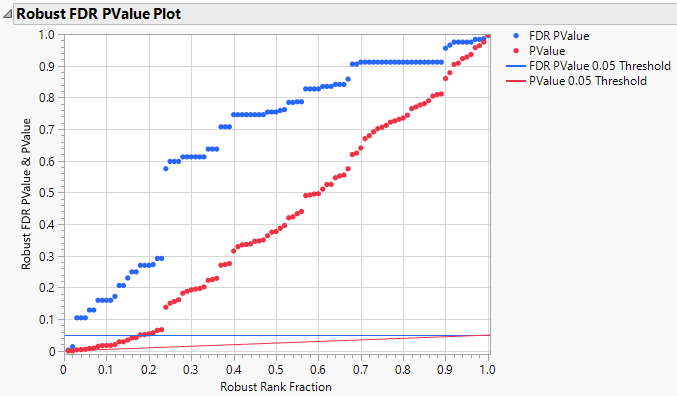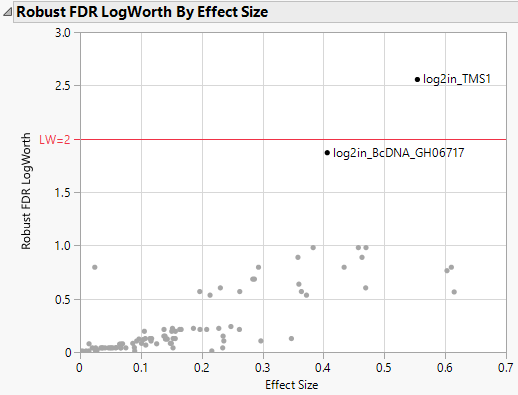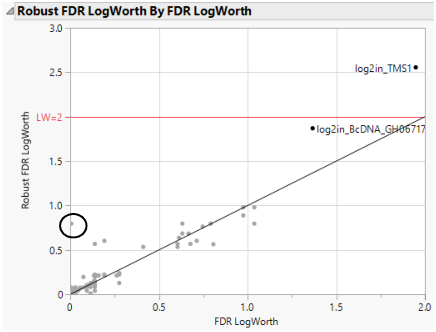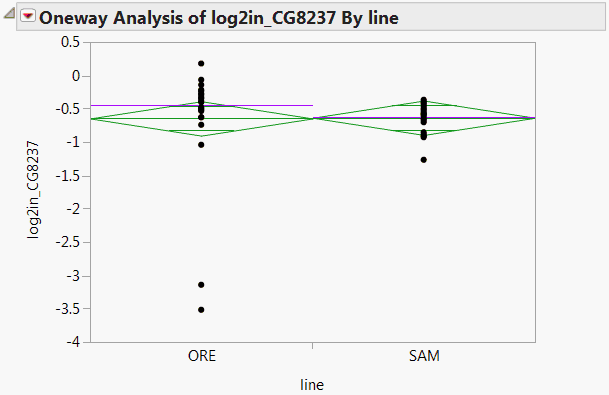Publication date: 04/12/2021

## Example of Robust Fit

1. Select Help > Sample Data Library and open Drosophila Aging.jmp.

2. Select Analyze > Screening > Response Screening.

3. Select all of the continuous columns and click Y, Response.

4. Select line and click X.

5. Check Robust.

6. Click OK.

Figure 22.14 Robust FDR PValue Plot for Drosophila DataNote that a number of tests are significant using the unadjusted robust p-values, as indicated by the red points that are less than 0.05. However, only two tests are significant according to the robust FDR p-values. These two points are more easily identified in a plot that shows FDR LogWorths.

7. Click the Robust FDR LogWorth by Effect Size disclosure icon.

8. Drag a rectangle around the point with a Robust FDR LogWorth value that exceeds 1.5.

9. In the graph, right click and select Row Label.

Figure 22.15 Robust LogWorth by Effect Size for Drosophila DataPoints above the red line at 2 have significance levels below 0.01 (-log10(0.01) = 2). A horizontal line at about 1.3 corresponds to a 0.05 significance level.

10. Click the Robust LogWorth by LogWorth disclosure icon.

Figure 22.16 Robust LogWorth by LogWorth for Drosophila DataIf the robust test for a response were identical to the usual test, its corresponding point would fall on the diagonal line in Figure 22.16. The circled point in the plot does not fall near the line, because it has a Robust LogWorth value that exceeds its LogWorth value.

11. Drag a rectangle around this point in the plot.

12. Find the row for this point in the Result table.

Note that the response, log2in_CG8237 has PValue 0.9568 and Robust PValue 0.0176.

13. Click the Response Screening red triangle and select Fit Selected Items.

A Fit Selected Items report is displayed containing a Oneway Analysis for the response log2in_CG8237. The plot shows two outliers for the ORE line (Figure 22.17). These outliers indicate why the robust test and the usual test give disparate results. The outliers inflate the error variance for the non-robust test, which makes it more difficult to see a significant effect. In contrast, the robust fit down-weights these outliers, thereby reducing their contribution to the error variance.

Figure 22.17 Oneway Analysis for log2in_CG8237Want more information? Have questions? Get answers in the JMP User Community (community.jmp.com).